# An intro to linear classification with Python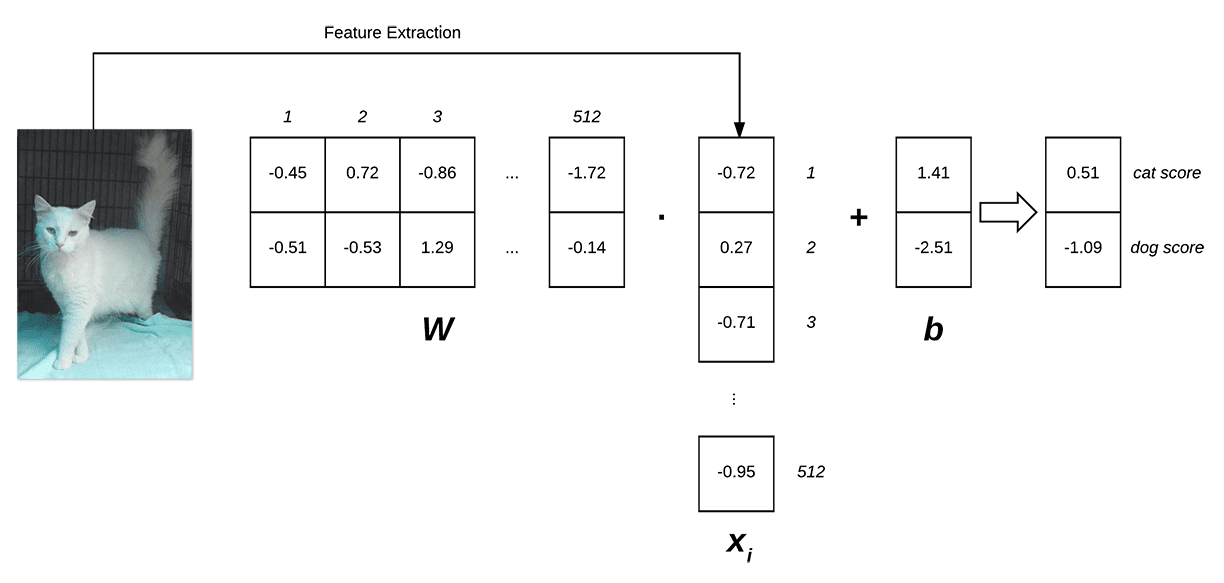Over the past few weeks, we’ve started to learn more and more about machine learning and the role it plays in computer visionimage classification, and deep learning.

We’ve seen how Convolutional Neural Networks (CNNs) such as LetNet can be used to classify handwritten digits from the MNIST dataset. We’ve applied the k-NN algorithm to classify whether or not an image contains a dog or a cat. And we’ve learned how to apply hyperparameter tuning to optimize our model to obtain higher classification accuracy.

However, there is another very important machine learning algorithm we have yet to explore — one that can be built upon and extended naturally to Neural Networks and Convolutional Neural Networks.

What is the algorithm?

It’s a simple linear classifier — and while it’s a straightforward algorithm, it’s considered the cornerstone building block of more advanced machine learning and deep learning algorithms.

Looking for the source code to this post?

## An intro to linear classification with Python

The first half of this tutorial focuses on the basic theory and mathematics surrounding linear classification — and in general — parameterized classification algorithms that actually “learn” from their training data.

From there, I provide an actual linear classification implementation and example using the scikit-learn library that can be used to classify the contents of an image.

### 4 components of parametrized learning and linear classifiers

I’ve used the word “parameterized” a few times now, but what exactly does it mean?

Simply put: parameterization is the process of defining the necessary parameters of a given model.

In the task of machine learning, parameterization involves defining our problem in terms of:

1. Data: This is our input data that we are going to learn from. This data includes both the data points (e.x., feature vectors, color histograms, raw pixel intensities, etc.) and their associated class labels.
2. Score function: A function that accepts our data as input and maps the data to class labels. For instance, given our input feature vectors, the score function takes these data points, applies some function f (our score function), and then returns the predicted class labels.
3. Loss function: A loss function quantifies how well our predicted class labels agree with our ground-truth labels. The higher level of agreement between these two sets of labels, the lower our loss (and higher our classification accuracy, at least on the training data). Our goal is to minimize our loss function, thereby increasing our classification accuracy.
4. Weight matrix: The weight matrix, typically denoted as W, is called the weights or parameters of our classifier that we’ll actually be optimizing. Based on the output of our score function and loss function, we’ll be tweaking and fiddling with the values of our weight matrix to increase classification accuracy.

Note: Depending on your type of model, there may exist many more parameters. But at the most basic level, these are the 4 building blocks of parameterized learning that you’ll commonly see.

Once we’ve defined these 4 key components, we can then apply optimization methods that allow us to find a set of parameters W that minimize our loss function with respect to our score function (while increasing classification accuracy on the data).

Next, we’ll look at how these components work together to build a linear classifier, transforming the input data into actual predictions.

### Linear classification: from images to labels

In this section, we are going to look at a more mathematical motivation of the parameterized model to machine learning.

To start we need our data. Let’s assume that our training dataset (either of images or extracted feature vectors) is denoted as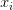$x_{i}$ where each image/feature vector has an associated class label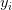$y_{i}$. We’ll assume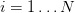$i = 1 \ldots N$ and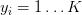$y_{i}= 1 \ldots K$ implying that we have N data points of dimensionality D (the “length” of the feature vector), separated into K unique categories.

To make this more concrete, consider our previous tutorial on using the k-NN classifier to recognize dogs and cats in images based on extracted color histograms.

This this dataset, we have N = 25,000 total images. Each image is characterized by a 3D color histogram with 8 bins per channel, respectively. This yields a feature vector with D = 8 x 8 x 8 = 512 entries. Finally, we know there are a total of K = 2 class labels, one for the “dog” class and another for the “cat” class.

Given these variables, we must now define a score function f that maps the feature vectors to the class label scores. As the title of this blog post suggests, we’ll be using a simple linear mapping: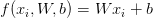$f(x_{i}, W, b) = Wx_{i} + b$

We’ll assume that each$x_{i}$ is represented as a single column vector with shape [D x 1]. Again, in this example, we’ll be using color histograms — but if we were utilizing raw pixel intensities, we can simply flatten the pixels of the image into a single vector.

Our weight matrix W has a shape of [K x D] (the number of class labels by the dimensionality of the feature vector).

Finally, b, the bias vector is of size [K x 1]. The bias vector essentially allows us to “shift” our scoring function in one direction or another, without actually influencing our weight matrix W — this is often critical for successful learning.

Going back to the Kaggle Dogs vs. Cats example, each$x_{i}$ is represented by a 512-d color histogram, so$x_{i}$ therefore has the shape [512 x 1]. The weight matrix W will have a shape of [2 x 512] and finally the bias vector b a size of [2 x 1].

Below follows an illustration of the linear classification scoring function f:Figure 1: Illustrating the dot product of weight matrix W and feature vector x, followed by addition of the bias term. (Inspired by Karpathy’s example in the CS231n course).

On the left, we have our original input image, which we extract features from. In this example, we’re computing a 512-d color histogram, but any other feature representation could be used (including the raw pixel intensities themselves), but in this case, we’ll simply use a color distribution — this histogram is our$x_{i}$ representation.

We then have our weight matrix W, which contains 2 rows (one for each class label) and 512 columns (one for each of the entries in the feature vector).

After taking the dot product between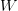$W$ and$x_{i}$, we add in the bias vector$b$, which has a shape of [2 x 1].

Finally, this yields two values on the right: the scores associated with the dog and cat labels, respectively.

Looking at the above equation, you can convince yourself that the input$x_{i}$ and$y_{i}$ are fixed and not something we can modify. Sure, we can obtain different$x_{i}$‘s by applying a different feature extraction technique — but once the features are extracted, these values do not change.

In fact, the only parameters that we have any control over are our weight matrix W and our bias vector b. Therefore, our goal is to utilize both our scoring function and loss function to optimize (i.e., modify) the weight and bias vectors such that our classification accuracy increases.

Exactly how we optimize the weight matrix depends on our loss function, but typically involves some form of gradient descent — we’ll be reviewing optimization and loss functions in a future blog post, but for the time being, simply understand that given a scoring function, we also define a loss function that tells us how “good” our predictions are on the input data.

### Advantages of parametrized learning and linear classification

There are two primary advantages to utilizing parameterized learning, such as in the approach I detailed above:

1. Once we are done training our model, we can discard the input data and keep only the weight matrix W and the bias vector bThis substantially reduces the size of our model since we only need to store two sets of vectors (versus the entire training set).
2. Classifying new test data is fastIn order to perform a classification, all we need to do is take the dot product of$W$ and$x_{i}$, followed by adding in the bias$b$. Doing this is substantially faster than needing to compare each testing point to every training example (as in the k-NN algorithm).

Now that we understand linear classification, let’s see how we can implement it in Python, OpenCV, and scikit-learn.

### Linear classification of images with Python, OpenCV, and scikit-learn

Much like in our previous example on the Kaggle Dogs vs. Cats dataset and the k-NN algorithm, we’ll be extracting color histograms from the dataset; however, unlike the previous example, we’ll be using a linear classifier rather than k-NN.

Specifically, we’ll be using a Linear Support Vector Machine (SVM) which constructs a maximum-margin separating hyperplane between data classes in an n-dimensional space. The goal of this separating hyperplane is to place all examples (or as many as possible, given some tolerance) of class i on one side of the hyperplane and then all examples not of class i on the other side of the hyperplane.

A detailed description of how Support Vector Machines work is outside the scope of this blog post (but is covered inside the PyImageSearch Gurus course).

In the meantime, simply understand that our Linear SVM utilizes a score function f similar to the one in the “Linear classification: from images to class labels” section of this blog post and then applies a loss function that is used to determine the maximum-margin separating hyperplane to classify the data points (again, we’ll be looking at loss functions in future blog posts).

To get started, open up a new file, name it linear_classifier.py , and insert the following code:

Lines 2-111 handle importing our required Python packages. We’ll be making use of the scikit-learn library, so if you do not already have it installed, make sure you follow these instructions to get it setup on your machine.

We’ll also be using my imutils Python package, a set of image processing convenience functions. If you do not already have imutils  installed, just let pip  install it for you:

We’ll now define our extract_color_histogram  function which will be used to extract and quantify the contents of our input images:

This function accepts an input image , converts it to the HSV color space, and then computes a 3D color histogram using the supplied number of bins  for each channel.

After computing the color histogram using the cv2.calcHist  function, the histogram is normalized and then returned to the calling function.

For a more detailed review of the extract_color_histogram  method, please refer to this blog post.

Next, let’s parse our command line arguments and initialize a few variables:

Lines 33-36 parse our command line arguments. We only need a single switch here, --dataset , which is the path to our input Kaggle Dogs vs. Cats dataset.

We then grab the imagePaths  to where each of the 25,000 images reside on disk, followed by initializing a data  matrix to store our extracted feature vectors along with our class labels .

Speaking of extracting features, let’s go ahead and do that:

On Line 47 we start looping over our input imagePaths . For each imagePath , we load the image  from disk, extract the class label , and then quantify the image by computing a color histogram. We then update our data  and labels  lists, respectively.

Currently, our labels  list is represented as a list of strings, either “dog” or “cat”. However, many machine learning algorithms in scikit-learn prefer that the labels  are encoded as integers, with one unique integer per class label.

Performing this conversion of class label string-to-integer is easy with the LabelEncoder  class:

After the .fit_transform  method is called, our labels  are now represented as a list of integers.

Our final code block will handle partitioning our data into training/testing splits, followed by training our Linear SVM and evaluating it:

Lines 70 and 71 handle constructing our training and testing split. We’ll be using 75% of our data for training and the remaining 25% for testing.

To train our Linear SVM, we’ll utilize the LinearSVC  implementation from the scikit-learn library (Lines 75 and 76).

Finally, we evaluate our classifier on Lines 80-82, displaying a nicely formatted classification report on how well our model performed.

One thing you’ll notice here is that I’m purposely not tuning hyperparameters here, simply to keep this example shorter and easier to digest. However, with that said, I leave tuning the hyperparameters of the LinearSVC  classifier as an exercise to you, the reader. Use our previous blog post on tuning the hyperparameters of the k-NN classifier as an example.

### Evaluating our linear classifier

1. The source code to this blog post using the “Downloads” section at the bottom of this tutorial.
2. The Kaggle Dogs vs. Cats dataset.

Once you have the code and dataset, you can execute the following command:

The feature extraction process should take approximately 1-3 minutes depending on the speed of your machine.

From there, our Linear SVM is trained and evaluated: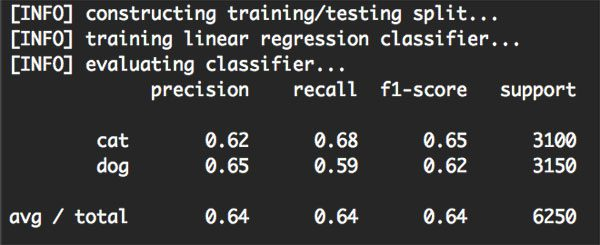Figure 2: Training and evaluating our linear classifier using Python, OpenCV, and scikit-learn.

As the above figure demonstrates, we were able to obtain 64% classification accuracy, or approximately the same accuracy as using tuned hyperparameters from the k-NN algorithm in this tutorial.

Note: Tuning the hyperparameters to the Linear SVM will lead to a higher classification accuracy — I simply left out this step to make the tutorial a little shorter and less overwhelming.

Furthermore, not only did we obtain the same classification accuracy as k-NN, but our model is much faster at testing time, requiring only a (highly optimized) dot product between the weight matrix and data points, followed by a simple addition.

We are also able to discard the training data after training is complete, leaving us with only the weight matrix W and the bias vector b, leading to a much more compact representation of the classification model.

## Summary

In today’s blog post, I discussed the basics of parameterized learning and linear classification. While simple, the linear classifier can be seen as the fundamental building blocks of more advanced machine learning algorithms, extending naturally to Neural Networks and Convolutional Neural Networks.

You see, Convolutional Neural Networks will perform a mapping of raw pixels to class labels similar to what we did in this tutorial — only our score function f will become substantially more complex and contain many more parameters.

A primary benefit of this parameterized approach to learning is that it allows us to discard our training data after our model has been trained. We can then perform classification using only the parameters (i.e., weight matrix and bias vector) learned from the data.

This allows classification to be performed much more efficiently since we: (1) do not need to store a copy of the training data in our model, like in k-NN and (2) we do not need to compare a test image to every training image (an operation that scales O(N), and can become quite cumbersome given many training examples).

In short, this method is significantly faster, requiring only a single dot product and an addition. Pretty neat, right?

Finally, we applied a linear classifier using Python, OpenCV, and scikit-learn to the Kaggle Dogs vs. Cats dataset. After extracting color histograms from the dataset, we trained a Linear Support Vector Machine on the feature vectors and obtained a classification accuracy of 64%, which is fairly reasonable given that (1) color histograms are not the best choice for characterizing dogs vs. cats and (2) we did not tune the hyperparameters to our Linear SVM.

At this point, we are starting to understand the basic building blocks that go into building Neural Networks, Convolutional Neural Networks, and Deep Learning models, but there is still a ways to go.

To start, we need to understand loss functions in more detail, and in particular, how the loss function is used to optimize our weight matrix to obtain more accurate predictions. Future blog posts will go into these concepts in more detail.

Before you go, be sure to sign up for the PyImageSearch Newsletter using the form below to be notified when new blog posts are published!If you would like to download the code and images used in this post, please enter your email address in the form below. Not only will you get a .zip of the code, I’ll also send you a FREE 17-page Resource Guide on Computer Vision, OpenCV, and Deep Learning. Inside you'll find my hand-picked tutorials, books, courses, and libraries to help you master CV and DL! Sound good? If so, enter your email address and I’ll send you the code immediately!

### 17 Responses to An intro to linear classification with Python

1.Sifan August 23, 2016 at 11:15 am #

Great tutorial and explanation,thank you! I want to use feature extractors like Sift,Surf or Hog instead of Color_histogram,can you show me how?thank you

•Adrian Rosebrock August 24, 2016 at 8:30 am #

I demonstrate how to use Histogram of Oriented Gradients as feature inputs to a Linear SVM inside Practical Python and OpenCV. To utilize SIFT/SURF or other keypoint detectors + local invariant descriptors you’ll need to construct a bag of visual words (BOVW) model. I discuss the BOVW model in detail (with lots of code examples) and apply it to image classification inside PyImageSearch Gurus. Be sure to take a look!

2.Rohan Saxena September 14, 2016 at 2:38 am #

First of all, being bored at work does pay well if you have a Smartphone and you browse through blogs.Amazing information with facts thoughtfully incorporated within. Definitely going to come back for more! 🙂

•Adrian Rosebrock September 15, 2016 at 9:35 am #

Thank you for the kind words Rohan! 🙂

3.Antoni October 18, 2016 at 4:54 am #

Now that I have train the model how do I test it

I mean with a different image from the ones i used and it isnt labeled like the ones in the data set

•Adrian Rosebrock October 20, 2016 at 8:59 am #

You would load your image from disk, pre-process it exactly like we did in this blog post, and call `model.predict` on it.

For what it’s worth, I demonstrate the basics of using machine learning classifiers with OpenCV + Python inside my book, Practical Python and OpenCV.

4.Anna_Maria May 30, 2017 at 2:22 am #

First I would like to thanks for your tutorial. Wonderful job and very useful source of information for newbies like me.
I want to use this classifier as a tool to extract background – water, from the images. I have to classes: water and others. Could you recommend me an approach/functions which could be the best? My idea is to based on the output I would like to give water black values, then remove unwanted objects from the image and find centroids of object of interest. The last part will be based on connecting components. Though I find it hard to mask the water. Thank you

•Adrian Rosebrock May 31, 2017 at 1:15 pm #

Instead of directly using machine learning methods to create pixel-wise segmentations of the image, have you considered using other segmentation methods such as thresholding, adaptive thresholding, GrabCut, etc.?

•Anna_Maria June 1, 2017 at 3:17 am #

Tbh I thought about it and already did it combining approaches with different color spaces. I have to do something with machine learning as it is my let say boss idea and I can’t do much about it

5.Anna_Maria May 31, 2017 at 12:19 am #

Hi Adrian. Thanks for the tutorial. It is a really big help for a newbie like me. I have a question could you recommend how, based on classification results, I can change pixel values of my two classes, to black for one and while to second class? Thanks

•Adrian Rosebrock May 31, 2017 at 1:03 pm #

Hi Anna Maria — I’m not sure what you mean by “based on classification results, I can change pixel values of my classes”? What exactly are you trying to accomplish?

•Anna Maria May 31, 2017 at 7:53 pm #

Hi Adrian. I wanted to use svm to perform classification over images with water and mixed(water and objects) and then change values of water into black and others into white. Extract background using svm

•Adrian Rosebrock June 4, 2017 at 6:31 am #

This sounds like a pixel-wise segmentation problem. Without seeing example images of what you’re working with, it’s hard for me to provide suggestions. You might want to consider researching pixel-wise segmentation using Random Forests. Deep learning can also be used here, but it’s likely overkill.

6.Jimmy Vivas July 9, 2018 at 9:18 am #

hello I have 1200 images that I need to cluster them based on their background color, could you tell me what algorithm would you recommend?

•Adrian Rosebrock July 10, 2018 at 8:25 am #

You could try extracting a color histogram and then applying the k-means algorithm.

1. - September 5, 2016

[…] couple weeks ago,we discussed the concepts of both linear classification and parameterized learning. This type of learning allows us to take a set of input data and class labels, and actually learn a […]

2. - October 10, 2016

[…] started with an introduction to linear classification that discussed the concept of parameterized learning, and how this type of learning enables us to […]